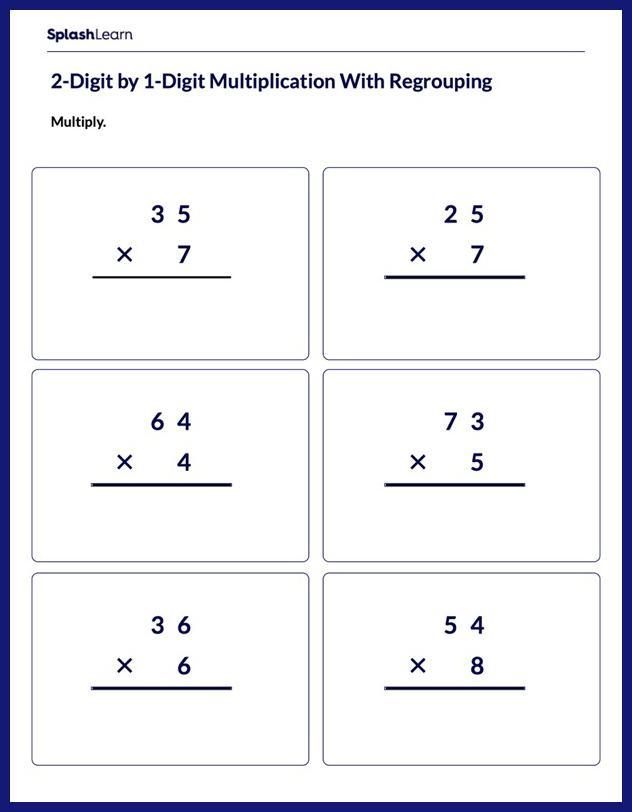# Multiply 2-Digit Number by 1-Digit Number Worksheet

Home > Multiply 2-Digit Number by 1-Digit NumberKids often develop misconceptions about concepts in mathematics, including multiplication. It is important to help them get over those misconceptions. The worksheet involves 2-digit and 1-digit numbers, it is important for students to gain confidence in a concept by working at different levels of complexity. Students will need to regroup to answer questions on multiplication.